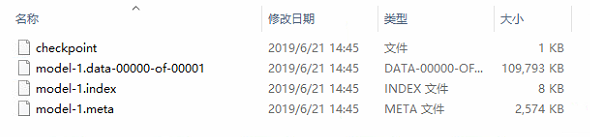# TensorFlow学习笔记之批归一化：tf.layers.batch_normalization()函数

tensorflow中的函数解析在这个博客——tf.nn.batch_normalization()函数解析

### 0 前言

y = γ ( x − μ ) / σ + β y=γ(x-μ)/σ+β

### 1 函数

tf.layers.batch_normalization(
inputs,
axis=-1,
momentum=0.99,
epsilon=0.001,
center=True,
scale=True,
beta_initializer=tf.zeros_initializer(),
gamma_initializer=tf.ones_initializer(),
moving_mean_initializer=tf.zeros_initializer(),
moving_variance_initializer=tf.ones_initializer(),
beta_regularizer=None,
gamma_regularizer=None,
beta_constraint=None,
gamma_constraint=None,
training=False,
trainable=True,
name=None,
reuse=None,
renorm=False,
renorm_clipping=None,
renorm_momentum=0.99,
fused=None,
virtual_batch_size=None,
)


• inputs：张量输入。
• axis：一个int，应该被规范化的轴（通常是特征轴）。例如，在使用data_format=“channels_first”Convolution2D层之后，在BatchNormalization中设置axis=1
• momentum：滑动平均值的动量。
• epsilon：小浮点数加上方差以避免被零除。
• center：如果为True，则将beta的偏移量添加到标准化张量。如果为False，则忽略beta
• scale：如果为True，则乘以gamma。如果为False，则不使用gamma。当下一层是线性的（例如，nn.relu）时，可以禁用此选项，因为可以由下一层进行缩放。
• beta_initializerbeta权重的初始值设定项。
• gamma_initializergamma权重的初始值设定项。
• moving_mean_initializer：滑动平均值的初始化器。
• moving_variance_initializer：滑动方差的初始值设定项。
• beta_regularizer：可选的beta权重正则化器。
• gamma_regularizergamma权重的可选调节器。
• beta_constraint：由Optimizer更新后应用于beta权重的可选投影函数（例如，用于实现层权重的规范约束或值约束）。函数必须将未投影的变量作为输入，并且必须返回投影的变量（必须具有相同的形状）。在进行异步分布式训练时，使用约束是不安全的。
• gamma_constraint：由Optimizer更新后应用于gamma权重的可选投影函数。
• training：要么是Python布尔值，要么是TensorFlow布尔值标量张量（例如占位符）。是以训练模式（使用当前批的统计数据进行规范化）还是以推理模式（使用滑动统计数据进行规范化）返回输出。注意：请确保正确设置此参数，否则您的训练 / 验证将无法正常工作。
• trainable：布尔值，如果为True，还将变量添加到图形集合GraphKeys.TRAINABLE_VARIABLES（请参见tf.variable）。
• name：字符串，层的名称。
• reuse：布尔值，是否以相同的名称重用前一层的权重。
• renorm：是否使用批量再规范化（https://arxiv.org/abs/1702.03275）。 这会在训练期间增加额外的变量。这个参数的任何一个值的推断都是相同的。
• renorm_clipping：一种字典，可以将关键字“rmax”、“rmin”、“dmax”映射到用于剪裁renorm校正的标量张量。校正（r，d）用作corrected_value = normalized_value * r + d，其中r被剪裁为[rmin，rmax]d被剪裁为[-dmax，dmax]。缺少的rmax、rmin和dmax分别设置为inf、0和inf。
• renorm_momentum：用renorm更新滑动方式和标准偏差的动量。与动量不同，这会影响训练，既不应太小（会增加噪音），也不应太大（会给出过时的估计）。注意，momentum仍然被用来得到均值和方差来进行推理。
• fused：如果False或者True，尽可能使用更快的融合实现。如果为False，则使用系统建议的实现。
• virtual_batch_size：一个int。默认情况下，virtual_batch_sizeNone，这意味着在整个批次中执行批次规范化。当virtual_batch_size不是None时，改为执行“Ghost Batch Normalization”，创建每个单独规范化的虚拟子批（使用共享gamma、beta和滑动统计）。必须在执行期间划分实际批大小。
• adjustment：仅在训练期间，采用包含输入张量（动态）形状的张量并返回一对（scalebias）以应用于标准化值（γβ之前）的函数。例如，如果axis=-1adjustment = lambda shape: ( tf.random_uniform(shape[-1:], 0.93, 1.07)tf.random_uniform(shape[-1:], -0.1, 0.1))将标准化值向上或向下缩放7%，然后将结果向上滑动0.1（每个功能都有独立的缩放和偏移，但在所有示例中都有共享），最后应用gamma 和/或 beta。如果没有，则不应用调整。如果指定了virtual_batch_size，则无法指定。

• 输出张量。

### 2 训练

(1)输入参数设置training=True

(2)计算loss时，要添加以下代码（即添加update_ops到最后的train_op中）。

net_optimizer = tf.train.AdamOptimizer(learning_rate=learning_rate)

update_ops = tf.get_collection(tf.GraphKeys.UPDATE_OPS)
with tf.control_dependencies(update_ops):
train_op = net_optimizer.minimize(loss)


### 3 验证

running_mean = momentum * running_mean + (1 - momentum) * sample_mean
running_std = momentum * running_std + (1 - momentum) * sample_std


output = (input - running_mean) / np.sqrt(running_std+eps)
output = trained_gamma * output + trained_beta


### 4 测试var_list = tf.trainable_variables()
saver = tf.train.Saver(var_list=var_list, max_to_keep=5)


### 5 批归一化

var_list = tf.trainable_variables()
g_list = tf.global_variables()
bn_moving_vars = [g for g in g_list if 'moving_mean' in g.name]
bn_moving_vars += [g for g in g_list if 'moving_variance' in g.name]
var_list += bn_moving_vars


### 6 总结

is_training = tf.placeholder(tf.bool)


...
...
[train_batch_image, train_batch_label] = sess.run([train_images, train_labels])
train_feed_dict = {x: train_batch_image,
y_: train_batch_label,
is_training: True}
...
...
[val_batch_image, val_batch_label] = sess.run([val_images, val_labels])
val_feed_dict = {x: val_batch_image,
y_: val_batch_label,
is_training: False}
...
...
`### 参考文章

11-22351707-12721
05-20713
07-033万+
08-041万+
04-30495
05-246万+
07-201071
12-151万+